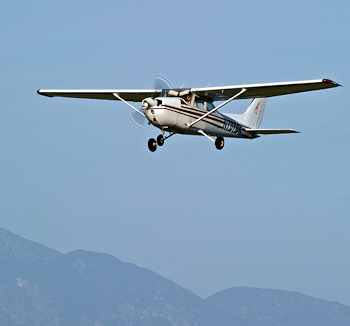Pilot's tip of the week

# Calculating Top Of Descent

Featuring

## Subscriber question:

"I always seem to be too high approaching the airport. Is there a simple way to plan my descent from cruise altitude to the pattern?" - Len M.

### Bob:“To figure out when to begin your descent, you need to know several things. These include your typical rate of descent, how many feet vertically you are above pattern altitude for the destination airport, and how much ground you’ll cover per minute at your current ground speed.

For example, at 90 knots you’ll cover 1-1/2 miles per minute, at 120 knots you’ll cover 2 miles per minute, and at 180 knots you’ll cover 3 miles per minute. To calculate when to begin the descent, start by subtracting pattern altitude from cruise altitude, then divide by the rate of descent. This will tell you how many minutes from the field you should begin your descent. Then you multiply the number of minutes by the number of miles you’ll cover per minute. This will tell you how far from the field you should begin your descent. So, let’s use these numbers:

• Cruise altitude = 10,000 feet MSL
• Pattern altitude = 1,000 feet MSL
• Ground speed = 120 knots
• Rate of descent = 500 feet/minute

To calculate the distance from the field for starting the descent, we’d first subtract pattern altitude from cruise altitude to determine how much altitude we have to lose. In this case, subtracting 1,000 feet from 10,000 feet tells us we need to lose 9,000 feet. Then, to determine how many minutes from the field we need to begin the descent, we divide the altitude we have to lose by the rate of descent in feet per minute. So, dividing 9,000 feet by a rate of descent of 500 feet per minute tells us we need to begin descending 18 minutes from the field. Then, to calculate the actual distance from the field where we should initiate the descent, we multiply minutes from the field by the miles per minute we’ll cover based on our current ground speed. In this example, we’d multiply 18 minutes x 2 miles per minute based on a groundspeed of 120 knots. That tells us we’d need to begin the descent 36 miles from the field.”

How do you decide when it's time to start descending from cruise altitude to your destination airport?

Next week's tip
Building IFR skills in VFR

## Get the Pilot’s Tip of the Week

#### Sign up here to receive tips like this every week along with videos, quizzes and more.

• This field is for validation purposes and should be left unchanged.